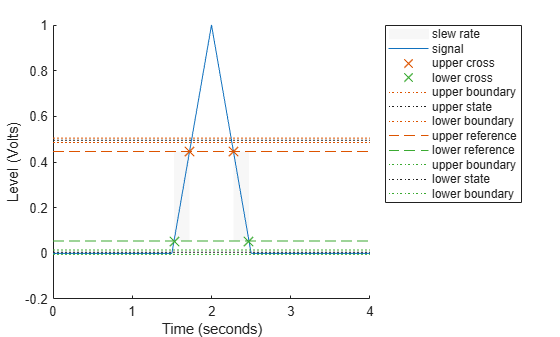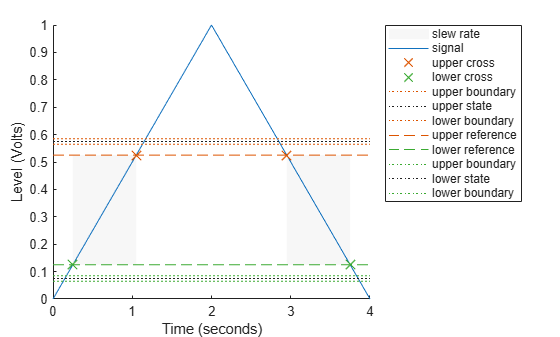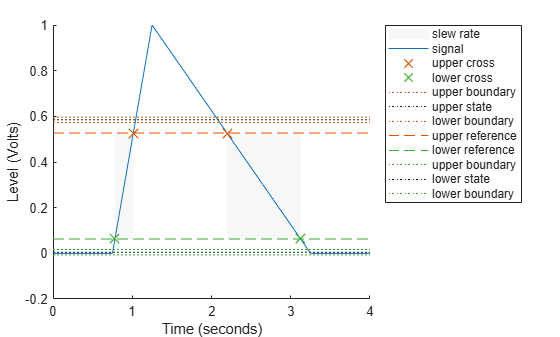Documentation

## Slew Rate of Triangular Waveform

This example shows how to use the slew rate as an estimate of the rising and falling slopes of a triangular waveform. Create three triangular waveforms. One waveform has rising-falling slopes of $±2$, one waveform has rising-falling slopes of $±\frac{1}{2}$, and one waveform has a rising slope of $+2$ and a falling slope of $-\frac{1}{2}$. Use `slewrate` to find the slopes of the waveforms.

Use `tripuls` to create a triangular waveform with rising-falling slopes of $±2$. Set the sampling interval to 0.01 seconds, which corresponds to a sample rate of 100 hertz.

```dt = 0.01; t = -2:dt:2; x = tripuls(t);```

Compute and plot the slew rate for the triangular waveform. Input the sample rate (100 Hz) to obtain the correct positive and negative slope values.

`slewrate(x,1/dt)````ans = 1×2 2.0000 -2.0000 ```

Change the width of the triangular waveform so it has slopes of $±\frac{1}{2}$. Compute and plot the slew rate.

```x = tripuls(t,4); slewrate(x,1/dt)``````ans = 1×2 0.5000 -0.5000 ```

Create a triangular waveform with a rising slope of $+2$ and a falling slope of $-\frac{1}{2}$. Compute the slew rate.

```x = tripuls(t,5/2,-3/5); s = slewrate(x,1/dt)```
```s = 1×2 2.0000 -0.5000 ```

The first element of `s` is the rising slope and the second element is the falling slope. Plot the result.

`slewrate(x,1/dt);`